# Couette Flow

Couette Flow is drag-induced flow either between parallel flat plates or between concentric rotating cylinders. Drag-induced flow is thus distinguished from pressure-induced flow, such as Poiseuille Flow. Flow between parallel flat plates is easier to analyze than flow between concentric cylinders. Thus, it is assumed that there is Laminar Flow of an incompressible Newtonian Fluid of density ρ and viscosity η between two parallel flat plates a constant distance H apart. The lower plate is of length L >> H and infinite width; it is stationary. The upper plate is of infinite length and infinite width; it moves at constant speed U in its own plane in the direction of the length of the lower plate. There is no variation in pressure p anywhere in the flow.
Because of the geometry, Couette flow is analyzed using rectangular or Cartesian coordinates (x, y). The x-direction is aligned with the direction of motion of the upper plate and the y-direction is aligned vertically upwards (see Figure 1). Because L >> H, Couette flow is fully-developed, that is the velocity u is independent of axial position x everywhere except near the ends of the stationary plate (at x = 0 and x = L). Solution of the mass and linear momentum Conservation Equations, specifically the Navier-Stokes equations, with boundary conditions of no-slip at both plates (y = 0 and y = H) yields [see Richardson (1989)]:
(1)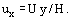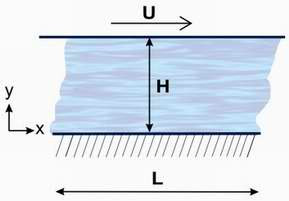Figure 1.
Thus, the axial velocity profile is linear (see Figure 2) and depends only on flow geometry and speed of the moving plate: it is independent of fluid properties. The mean axial velocity ūx is given by:
(2)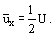The volumetric flow rate per unit width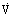between the plates is given by:
(3)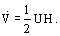Practical realization of Couette flow between parallel flat plates is difficult. A good approximation can, however, be obtained in the annular gap between two rotating concentric cylinders of slightly differing radii. Thus, if the inner cylinder has an outer radius R1 and the outer cylinder has an inner radius R2, the flow between them is very similar to that given by Eq. (1) provided that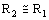. Flow between rotating concentric cylinders is susceptible to a centrifugal instability called the Taylor Instability [see Chandrasekhar (1961) and Drazin & Reid (1981)], which occurs when the inner cylinder is rotated and the outer cylinder is kept stationary and the Taylor Number Ta defined by:
(4)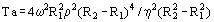exceeds a critical value Tac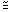1700, where ω denotes the angular velocity of the inner cylinder. Flow between rotating concentric cylinders forms the basis of the Couette viscometer. In order to avoid the Taylor instability, it is usual to rotate the outer cylinder and keep the inner cylinder stationary. Then, if there is a torque or moment F x r on the inner cylinder, it can be shown that [see Fredrickson (1964)]:
(5)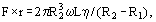where ω denotes the angular velocity of the outer cylinder and L the length of the inner cylinder. Measurement of F × r, ω, L, R1 and R2 permits evaluation of η. Note, incidentally, that Eq. (5) makes no allowance for end effects; these can, however, be rendered small by making L >> R2 > R1.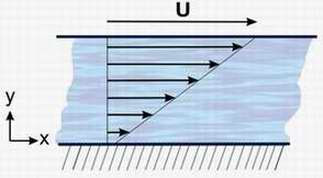Figure 2.